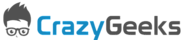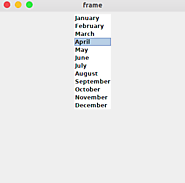Updated by sriniwas pawar on Oct 09, 2019
REPORTsriniwas pawar
Owner
9 items   1 followers   0 votes   0 views

# Java Programming Important Methods

Java Programming Important Methods

1

## Java Math abs() method with examples - CrazyGeeksIn Java, abs() function returns the absolute value of the given argument. By using abs function you can return absolute value for an int, float, double, short, long, byte etc. data types.

2

## length and length() in Java - CrazyGeekslength and length in Java are used to check the length of the Array and the String. The main difference between length and length() is given below:

3

## Java String Substring - CrazyGeeksThe substring() method in Java returns the substring of the given string. You can pass indexes from which position the substring should be started and ended. The substring() method in Java has two variants. In this tutorial, you will learn both variants with examples.

4

## Insertion Sort in Java - CrazyGeeksInsertion Sort is the simple algorithm for sorting elements of small datasets. In Insertion sort algorithm, the array element is removed and then located at the position it belongs by comparing values. As the complexity of this algorithm is O(n*2) where n is the number of elements. You can not use this algorithm for large datasets. In this article, you are going to learn the Insertion sort algorithm program in Java.

5

## Java Convert Integer to String - CrazyGeeksThere are different ways of converting int to string by using different available methods. In this tutorial, we will learn some of them below.

6

## Java Stack Class Tutorial with Examples - CrazyGeeksThe stack is a subclass of vector class in Java. It basically based on LIFO structure that is Last In First Out structure. It has two methods and they are push and pop but as it extends Vector class it gets three more methods search, peek and empty method. By using these methods you can do insert, remove, search etc. operation.

7

## Java JList Basics with Examples - CrazyGeeksJList is used to display an array of vectors in Java. JList comes with Swing package and also it inherits JComponent Class. JList displays a set of elements

8

## Bubble Sort in Java - CrazyGeeksBubble Sort is the simplest algorithm for sorting elements. In the Bubble sort algorithm, the array is traversed by comparing the current element with the next element each time. If the current element is greater than the next element then the positions will be swapped in this way the array will be sorted. As the complexity of this algorithm is Ο(n2) where n is the number of elements. This algorithm can not be used for large datasets. In this article, you are going to learn the Bubble sort algorithm program in Java.

9

## Binary Search in Java - CrazyGeeksFor the binary search program in Java if the array values are not defined already it will ask the user first to enter the size of the array. Then the user will enter array elements one by one. After adding all the elements to array ask the user to enter the element to search in an array by using binary search.